Related Articles

# Filtering a PySpark DataFrame using isin by exclusion

• Last Updated : 29 Jun, 2021

In this article, we will discuss how to filter the pyspark dataframe using isin by exclusion.

isin(): This is used to find the elements contains in a given dataframe, it takes the elements and gets the elements to match the data.

Syntax: isin([element1,element2,.,element n)

Creating Dataframe for demonstration:

## Python3

 `# importing module``import` `pyspark`` ` `# importing sparksession from pyspark.sql module``from` `pyspark.sql ``import` `SparkSession`` ` `# creating sparksession and giving an app name``spark ``=` `SparkSession.builder.appName(``'sparkdf'``).getOrCreate()`` ` `# list  of students  data  with null values``# we can define null values with none``data ``=` `[[``1``, ``"sravan"``, ``"vignan"``],``        ``[``2``, ``"ramya"``, ``"vvit"``],``        ``[``3``, ``"rohith"``, ``"klu"``],``        ``[``4``, ``"sridevi"``, ``"vignan"``],``        ``[``5``, ``"gnanesh"``, ``"iit"``]]`` ` `# specify column names``columns ``=` `[``'ID'``, ``'NAME'``, ``'college'``]`` ` `# creating a dataframe from the lists of data``dataframe ``=` `spark.createDataFrame(data, columns)``dataframe.show()`

Output: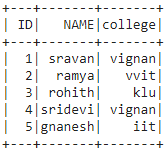Method 1: Using filter()

filter(): This clause is used to check the condition and give the results, Both are similar

Syntax: dataframe.filter(condition)

Example 1: Get the particular ID’s with filter() clause

## Python3

 `# get the ID : 1,2,3 from dataframe``dataframe.``filter``((dataframe.``ID``).isin([``1``,``2``,``3``])).show()`

Output: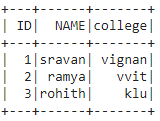Example 2: Get names from dataframe columns.

## Python3

 `# get name as sravan``dataframe.``filter``((dataframe.NAME).isin([``'sravan'``])).show()`

Output: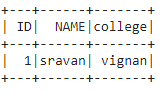Method 2: Using Where()

where(): This clause is used to check the condition and give the results

Syntax: dataframe.where(condition)

Example 1: Get the particular colleges with where() clause.

## Python3

 `# get college as vignan``dataframe.where((dataframe.college).isin([``'vignan'``])).show()`

Output: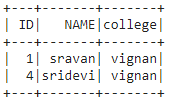Example 2: Get ID except 5 from dataframe.

## Python3

 `# get ID except 1``dataframe.where(~(dataframe.``ID``).isin([``1``])).show()`

Output: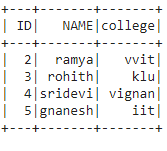Attention geek! Strengthen your foundations with the Python Programming Foundation Course and learn the basics.

To begin with, your interview preparations Enhance your Data Structures concepts with the Python DS Course. And to begin with your Machine Learning Journey, join the Machine Learning – Basic Level Course

My Personal Notes arrow_drop_up